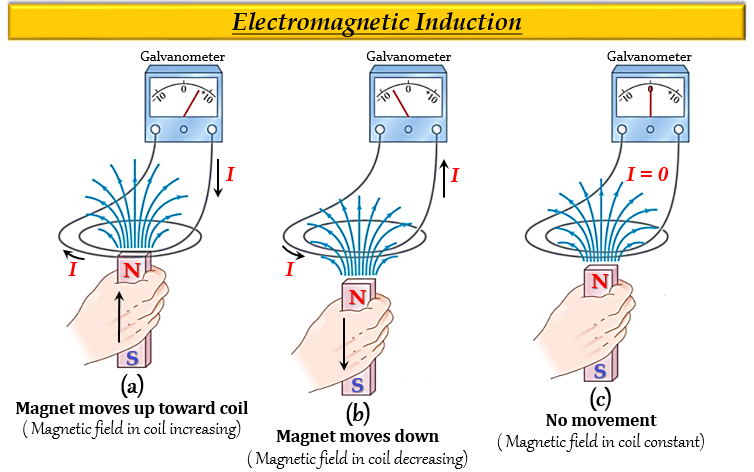# The phenomenon of electromagnetic induction is$(a)$. the process of charging a body.$(b)$. the process of generating magnetic field due to a current passing through a coil.$(c)$. producing induced current in a coil due to relative motion between a magnet and the coil.$(d)$. the process of rotating a coil of an electric motor.

The correct answer is:  $(c)$ producing induced current in a coil due to relative motion between a magnet and the coil.

Electromagnetic induction:

The change in magnetic field lines in a coil is the cause of induced electric current in it, and this phenomenon is known as Electromagnetic induction.

Explanation

Electromagnetic Induction is a process where a conductor placed in a changing magnetic field $(or\ a\ conductor\ moving\ through\ a\ stationary\ magnetic\ field)$ causes the production of an Electro-Motive Force $(Voltage)$ across the electrical conductor.

The process of electromagnetic induction works in reverse as well, so that a moving electrical charge generates a magnetic field.

The below diagram shows the experiment of electromagnetic induction with the help of a galvanometer, a coil, and a magnet.

• When the magnet is moved up toward the coil, the magnetic field increases which shows deflection in the needle of the galvanometer.
• When the magnet moves down, the magnetic field in the coil decreases which can be observed as a deflection in the galvanometer.
• When the magnet is not moved, there is no deflection observed in the galvanometer.